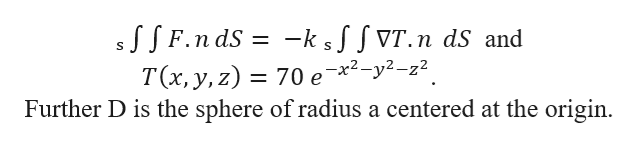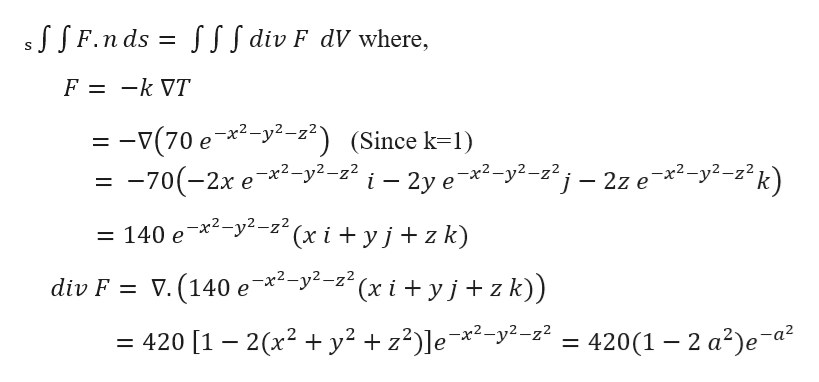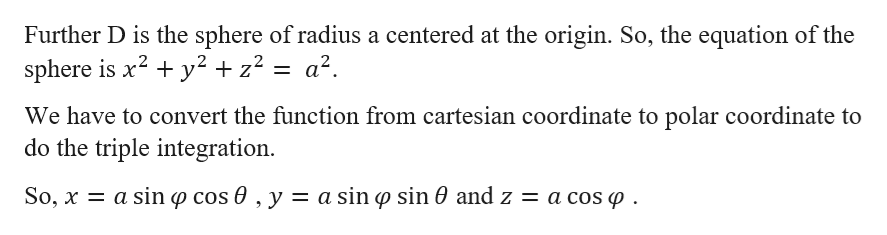Fourier's Law of heat transfer​ (or heat​ conduction) states that the heat flow vector F at a point is proportional to the negative gradient of the​ temperature: that​ is,F=−k∇​T,which means that heat energy flows from hot regions to cold regions. The constant k is called the​ conductivity, which has metric units of​J/m-s-K or​ W/m-K. A temperature function T for a region D is given below. Find the net outward heat flux. A temperature function for a region D is given below Find the net outward heat flux dS∫∫S  F•n dS=−k∫∫S  ∇T•n dSacross the boundary S of D. It may be easier to use the Divergence Theorem and evaluate a triple integral. Assume thatk=1. ​T(x,y,z)=70 e-x^2-y^2-z^2    D is the sphere of radius a centered at the origin.

Question
Fourier's Law of heat transfer​ (or heat​ conduction) states that the heat flow vector F at a point is proportional to the negative gradient of the​ temperature: that​ is,
F=−k∇​T,
which means that heat energy flows from hot regions to cold regions. The constant k is called the​ conductivity, which has metric units of​J/m-s-K or​ W/m-K. A temperature function T for a region D is given below. Find the net outward heat flux. A temperature function for a region D is given below Find the net outward heat flux
dS∫∫S  Fn dS=−k∫∫S  ∇T•n dS
across the boundary S of D. It may be easier to use the Divergence Theorem and evaluate a triple integral. Assume that
k=1.

​T(x,y,z)=70 e-x^2-y^2-z^2
D is the sphere of radius a centered at the origin.
Step 1

Given that,help_outlineImage TranscriptioncloseF.n dS -ks^fVT.n dS and 70 е *2-у2-2? Further D is the sphere of radius a centered at the origin T(x, y,z) fullscreen
Step 2

We want to use the Divergence Theorem and evaluate a triple integral.

We know,help_outlineImage TranscriptioncloseJSF.n ds S SIf div F dV where, S F = -k VT = -v(70 e *-y2-22) (Since k1) -70(-2x e2-y2-z2 i - 2y ex2-y2-z2j - 2z e *2-y2-z2k) = 140 e*-y--z(x i + yj+ z k) div F V. (140 e*2-y2-z"(x i + yj + z k)) = 420 [1 - 2(x2 y2 z2)]e*2-y2--22 = 420(1 -2 a2)e a2 fullscreen
Step 3help_outlineImage TranscriptioncloseFurther D is the sphere of radius a centered at the origin. So, the equation of the sphere is x2 y2 +z2 = a2. We have to convert the function from cartesian coordinate to polar coordinate to do the triple integration So, x a sinp cos 0 , y = a sin p sin 0 and z = a cos p fullscreen

Want to see the full answer?

See Solution

Want to see this answer and more?

Our solutions are written by experts, many with advanced degrees, and available 24/7

See Solution
Tagged in

Calculus